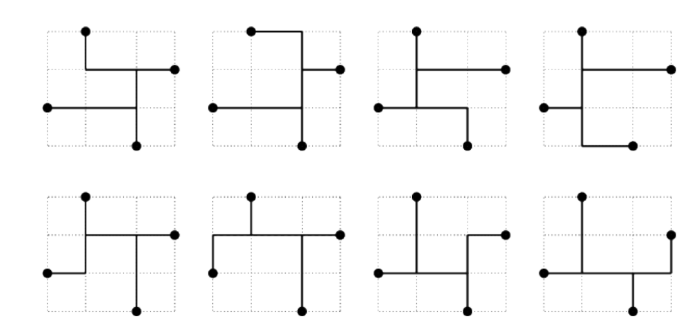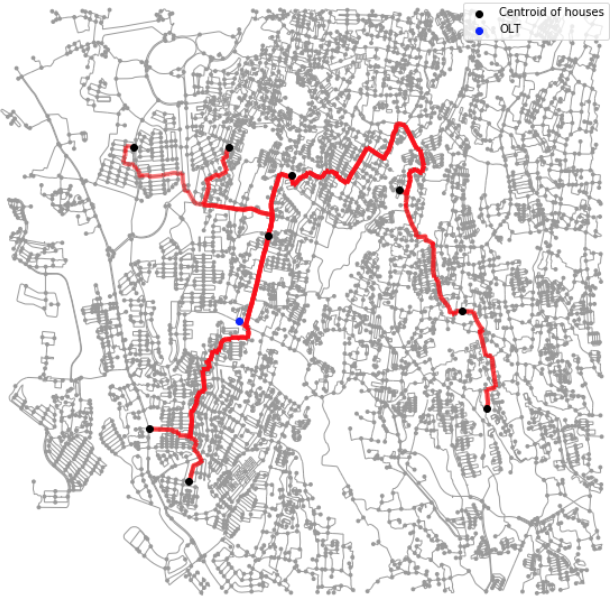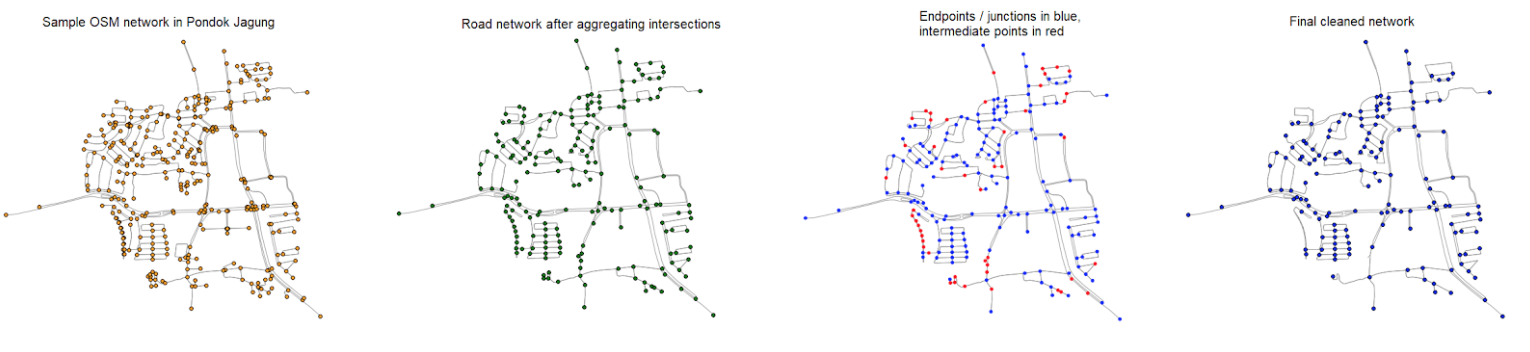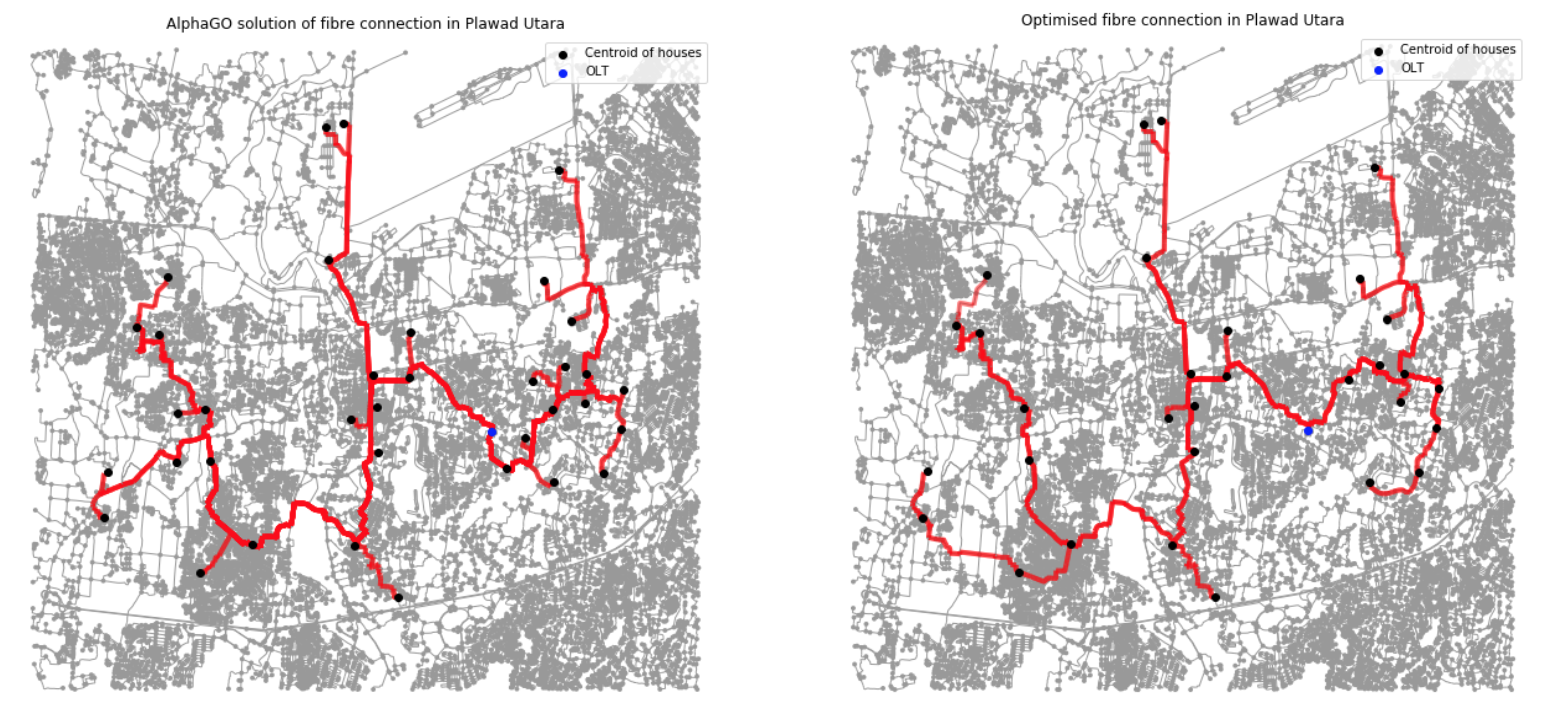## The Objective

Maximizing CLV from new fibre deployments at shortest route within an optimal budget

### The Challenge - Steiner Tree Problem

Given an undirected graph with non-negative edge weights and a subset of vertices, usually referred to as terminals, the Steiner tree problem in graphs requires a tree of minimum weight that contains all terminals.

Connecting OLTs to the FDTs can be viewed as a Steiner tree problem. Other known algorithms are super slow and their runtime scales exponentially in their input size.

This can be difficult because there are so many possibilities to try. The diagram below shows a few possible ways to connect four points. And these are just a few examples on a very small problem!### Steiner Tree vs Alpha GO

Steiner TreeLinear programming / heuristic
10 seconds
Sub-optimal, obvious mistakes

Alpha GONeural network

1.5 hours

Near optimal

## The Solution

To first achieve a digital mapping of the road network, we:

• Modelled the open street road network as a graph with each point as a vertex and road network as an edge
• Aggregate road intersections to simplify graph and treat all roads as bi-directional
• Removed intermediate points which are non-essential
• Mapped each centroid and OLT to the closest vertexNext we applied an Artificial Neural Network (ANN) supported random tree search algorithm (AlphaGo) to optimize the fibre cables routing layout.

## The Outcome

• Achieve up to 30% shorter routes
• Reduce the total cost spent on the feeder cable by approximately 30%.